{{ message }}
/ bokeh Public

# [BUG] Behaviour of automated padding for ranges is inconsistent#9724

Closed
opened this issue Feb 24, 2020 · 24 comments · Fixed by #9789
Closed

# [BUG] Behaviour of automated padding for ranges is inconsistent#9724

opened this issue Feb 24, 2020 · 24 comments · Fixed by #9789
Labels
Milestone

###Tikonderoga commented Feb 24, 2020 • edited

 Exists in Bokeh from 0.13.0 till 1.4.0 Tested on Windows 10 and Ubunto 16.0, on Firefox and Chrome (most recent versions). That's the weird bug, so explanation will be rather long. Example 1: ``````from bokeh.plotting import figure, output_file, show x = [0.1, 0.5, 1.0, 1.5, 2.0, 2.5, 3.0] y1 = [30 for xx in x] legend_items = [] p = figure(plot_width=500, plot_height=400) l1 = p.line(x, y1, line_width=2, color='red') p.y_range.start = 0 li1 = LegendItem(label='Line 1', renderers=[l1]) legend_items.append(li1) legend = Legend(items=legend_items, location=(0, -30)) legend.click_policy = "hide" p.add_layout(legend, 'left') show(p) `````` That produces pretty looking chart: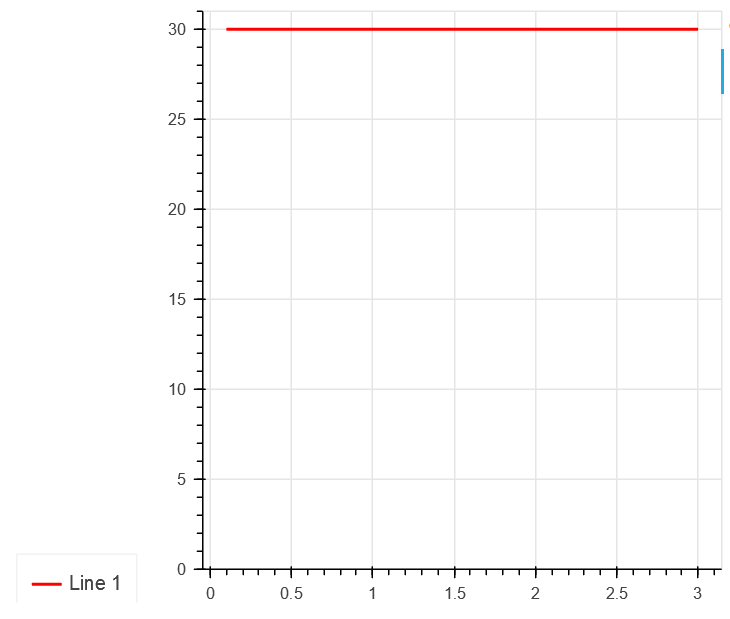Automatic padding added a little bit of space between horizontal line and top end of the chart, so line is clearly visible. Example 2: ``````from bokeh.plotting import figure, output_file, show x = [0.1, 0.5, 1.0, 1.5, 2.0, 2.5, 3.0] y1 = [300 for xx in x] legend_items = [] p = figure(plot_width=500, plot_height=400) l1 = p.line(x, y1, line_width=2, color='red') p.y_range.start = 0 li1 = LegendItem(label='Line 1', renderers=[l1]) legend_items.append(li1) legend = Legend(items=legend_items, location=(0, -30)) legend.click_policy = "hide" p.add_layout(legend, 'left') show(p) ``````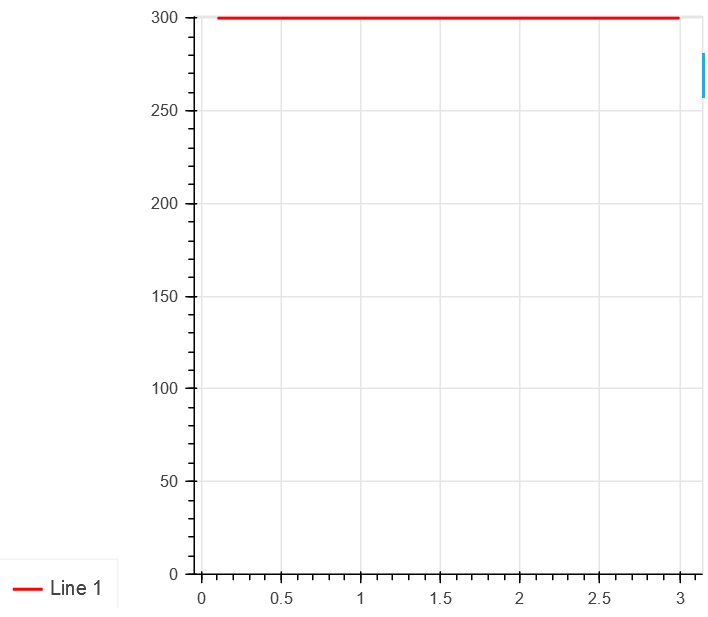With line located at Y=300, there's no automatic padding added, so line located directly on the border of the chart. One can argue, it can be easily fixed bu using customized y_range, but manually setting y_range bounds makes it hard to use new feature from 1.4.0 (#9144): Example 3 (only works in Bokeh 1.4.0): ``````from bokeh.plotting import figure, output_file, show x = [0.1, 0.5, 1.0, 1.5, 2.0, 2.5, 3.0] y1 = [30 for xx in x] y2 = [60 for xx in x] y3 = [90 for xx in x] legend_items = [] # create a new plot with a log axis type p = figure(plot_width=500, plot_height=400) l1 = p.line(x, y1, line_width=2, color='red') l2 = p.line(x, y2, line_width=2, color='blue') l3 = p.line(x, y3, line_width=2, color='green') p.y_range.start = 0 li1 = LegendItem(label='Line 1', renderers=[l1]) li2 = LegendItem(label='Line 2', renderers=[l2]) li3 = LegendItem(label='Line 3', renderers=[l3]) legend_items.append(li1) legend_items.append(li2) legend_items.append(li3) p.y_range.only_visible = True legend = Legend(items=legend_items, location=(0, -30)) legend.click_policy = "hide" p.add_layout(legend, 'left') show(p) `````` Now we are using automated Y-range scaling. All lines are clearly visible no matter how many lines we have hidden: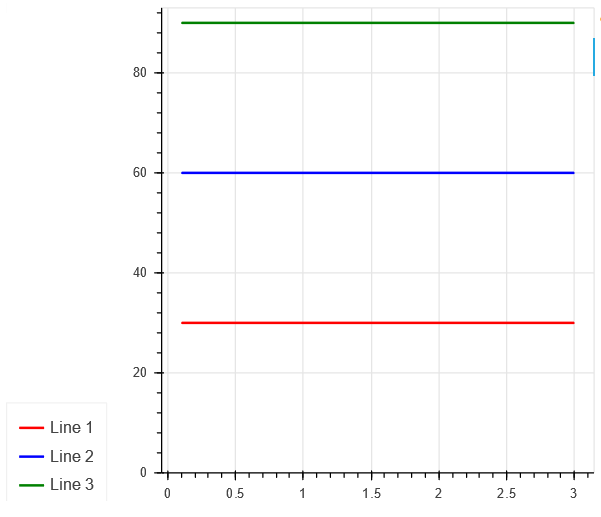or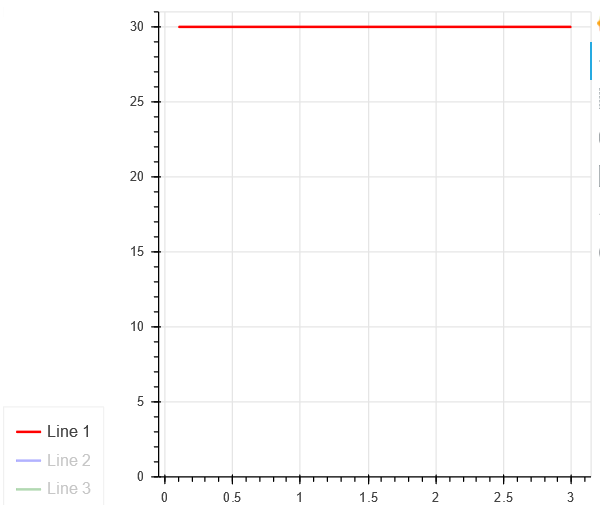But if we change Y-values for lines back to 300... Example 4 (only works in Bokeh 1.4.0): ``````from bokeh.plotting import figure, output_file, show x = [0.1, 0.5, 1.0, 1.5, 2.0, 2.5, 3.0] y1 = [300 for xx in x] y2 = [600 for xx in x] y3 = [900 for xx in x] legend_items = [] # create a new plot with a log axis type p = figure(plot_width=500, plot_height=400) l1 = p.line(x, y1, line_width=2, color='red') l2 = p.line(x, y2, line_width=2, color='blue') l3 = p.line(x, y3, line_width=2, color='green') p.y_range.start = 0 li1 = LegendItem(label='Line 1', renderers=[l1]) li2 = LegendItem(label='Line 2', renderers=[l2]) li3 = LegendItem(label='Line 3', renderers=[l3]) legend_items.append(li1) legend_items.append(li2) legend_items.append(li3) p.y_range.only_visible = True legend = Legend(items=legend_items, location=(0, -30)) legend.click_policy = "hide" p.add_layout(legend, 'left') show(p) `````` For 3 lines it works fine: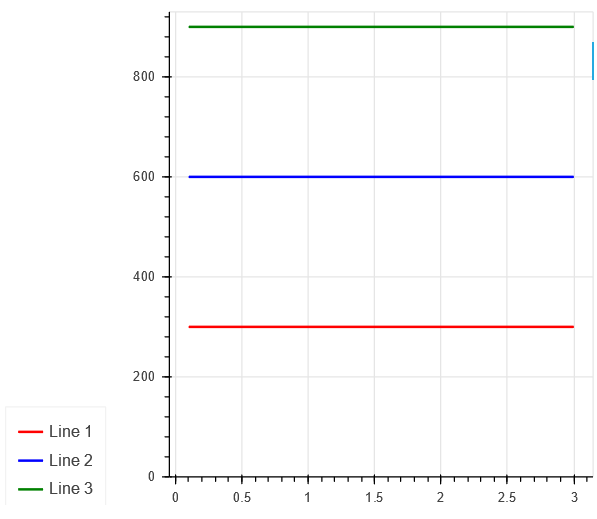For 2 lines it works fine: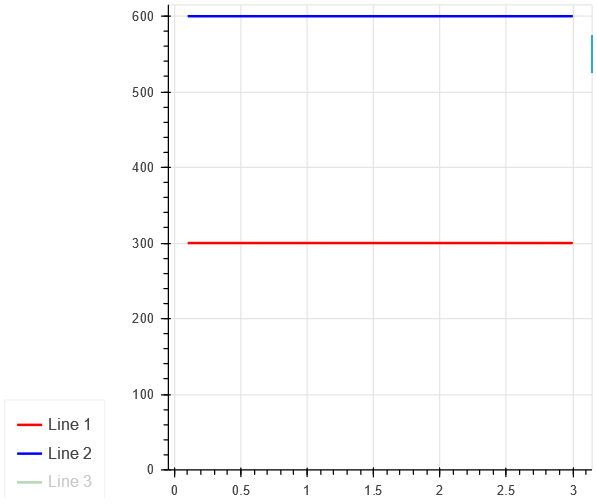But with only 1 line visible automated padding not working again: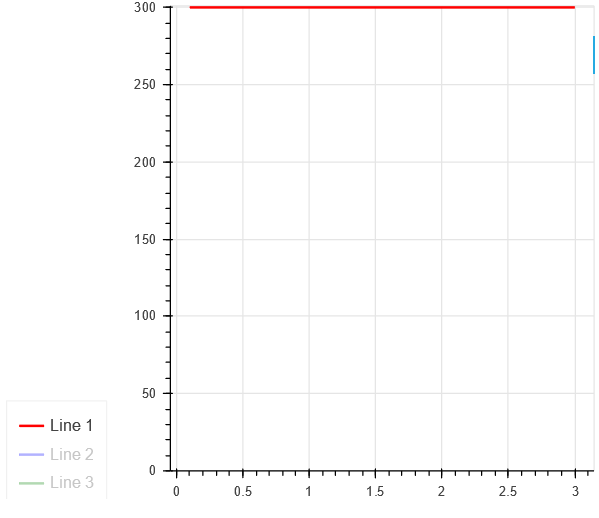What I would expect? I would expect to see similar gap between red line and top boundary of the chart, as I see for blue and green lines. Why it's important? Without callbacks there's no way to change y_range for the chart when certain lines became hidden and visible. As far as I understand, #9144 was supposed to provide simple way to do so, but since automated padding not always working the way you expect it to work, it's almost useless. The text was updated successfully, but these errors were encountered:

###bryevdv commented Feb 24, 2020 • edited

 @Tikonderoga can you please try and report back with 2.0.0rc1 (available on pypi or bokeh/dev channel on anaconda.org) Please note the starting with 2.0 hiding glyphs in the legend will remove them from automatic ranging computations (often requested feature) Also, AFAICT, a summary of the issue is this, please confirm: When one end of a DataRange1d is set explicitly (e.g. `p.y_range.start = 0`) the other end stops applying padding (but should not stop).

###Tikonderoga commented Feb 24, 2020 • edited

 @bryevdv Sorry, as I mentioned, it's weird issue and my description was lacking. Let me try it again, using your template. Issue (exists for all versions of Bokeh?): When one end of a DataRange1d is set explicitly (e.g. p.y_range.start = 0) the automatic padding for other end does not scale well (see the difference in padding for different Y-ranges in next screen).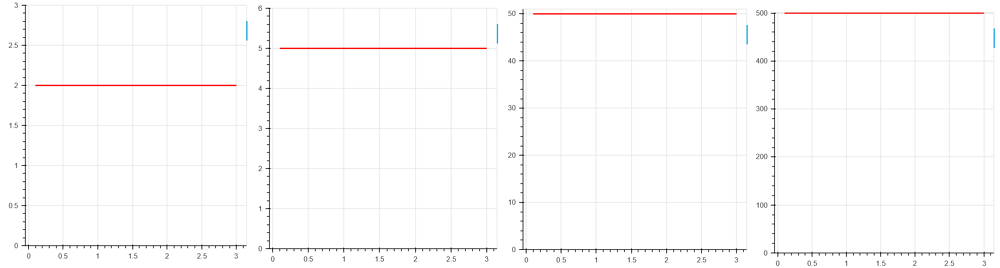In Bokeh > 1.4.0 is manifests itself in a case when p.y_range.only_visible = True: Y-range is large, breaks when 1 line visible: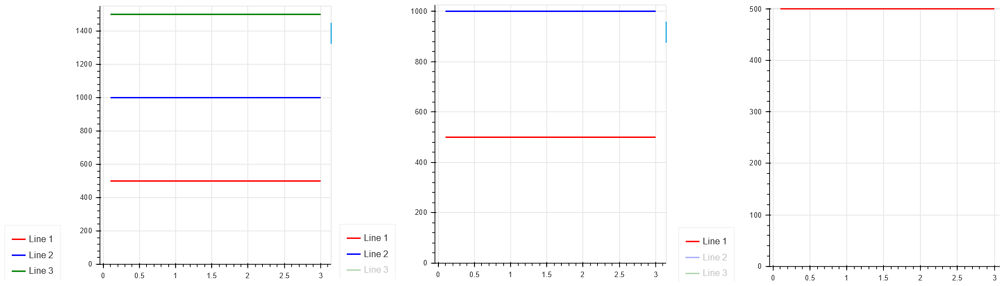I think there's something wrong in a way how automatic padding is being calculated - it's looks like it's not taking size of range into consideration. And when you have only one line visible out of many, same code path is being used.

###bryevdv commented Feb 26, 2020 • edited

 @Tikonderoga I have a suspicion, but have not yet been able to look into it. You could check out the BokehJS for DataRange1d in the mean time, if you like. My suspicion is this: the default padding units are percentage the percentage is always applied to the computed auto-range, even if one end is fixed the computed auto-range with a straight line is zero therefore the computed padding is zero (a percentage of zero) If this is actually the case, I am not sure I could conclude that the observed behavior is not, in fact, the correct behavior. I would not be in favor of adding magic, implicit special cases. So what could be done? Some ideas: Nothing. In cases like this (which don't seem like they would be common), absolute padding units are an alternative option Add a "min_padding" threshold that is always and uniformly applied (this avoids hidden magic for this one special case)

###bryevdv commented Feb 26, 2020 • edited

 Looking quickly at the code, I do believe this is the case, and as I mentioned above this behavior not inconsisten, it is in fact completely consistent: the padding is always a percentage of the computed min/max range, even if that range is zero. For now, changing `range_padding_units` is an option. If you are interested to work up a PR to add a new `min_padding` property, then I would be happy to help out. Otherwise, I am inclined to close this with `noaction` since it is a pretty obscure corner case.

###Tikonderoga commented Feb 26, 2020 • edited

 @bryevdv First of all, I would still argue it's inconsistent: Look at the image below. For all lines, at any Y-value, computed min/max range would be zero. Why there's padding added for lines A, B and C, but not for D?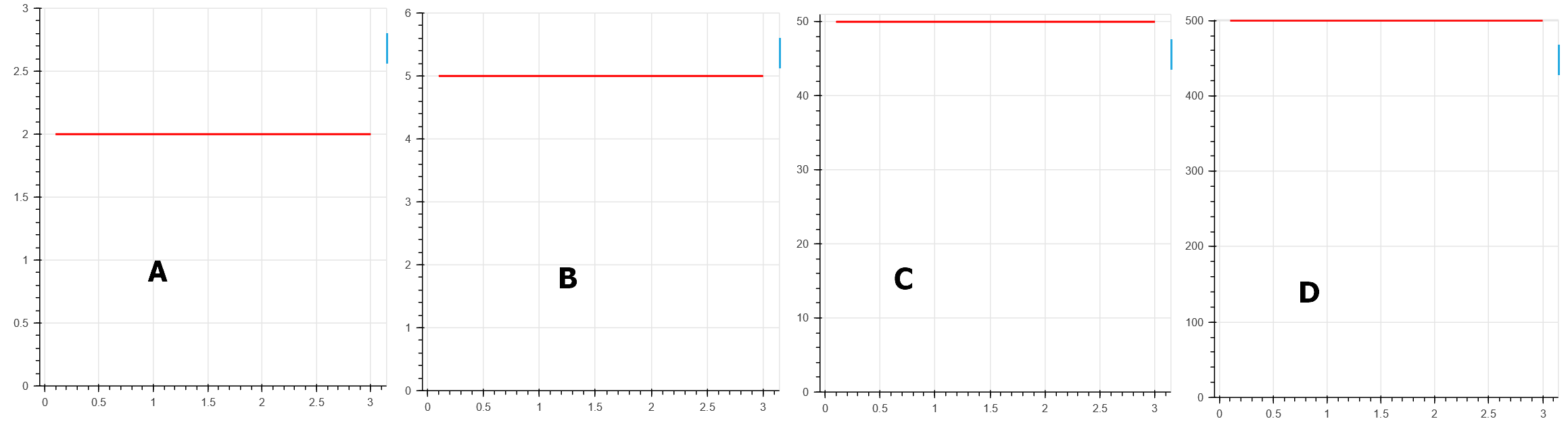Also, which part of Bokeh code should I look into? Is it bokeh/bokehjs/src/lib/models/ranges/data_range1d.ts ?

###bryevdv commented Feb 26, 2020 • edited

 It's not really possible for me to speculate with just the images. Are there other invisible glyphs? What is the configuration of the data range? For that matter, are the "ABCD" labels or text glyphs, or were they added outside Bokeh after the fact completely? Code for what you have above would help clarify. Is it bokeh/bokehjs/src/lib/models/ranges/data_range1d.ts Yes that's the main file of interest. The range will ask any available renderers for their bounds (and then union them together), so there's also some possibility that the bounds computation in the `Line` glyph is also relevant.

###Tikonderoga commented Feb 26, 2020

 @bryevdv Sure, I'll provide code and a lot of test cases where padding (both manual and automatic) appears to be broken. There will be multiple comments Case 1. ``````from bokeh.plotting import figure, output_file, show from bokeh.layouts import gridplot from bokeh.models import ColumnDataSource, Range1d, LabelSet, Label x = [0.1, 0.5, 1.0, 1.5, 2.0, 2.5, 3.0] y1 = [2 for xx in x] y2 = [5 for xx in x] y3 = [50 for xx in x] y4 = [100 for xx in x] y5 = [500 for xx in x] p1 = figure(plot_width=300, plot_height=300) l1 = p1.line(x, y1, line_width=2, color='red') p1.y_range.start = 0 p2 = figure(plot_width=300, plot_height=300) l2 = p2.line(x, y2, line_width=2, color='red') p2.y_range.start = 0 p3 = figure(plot_width=300, plot_height=300) l3 = p3.line(x, y3, line_width=2, color='red') p3.y_range.start = 0 p4 = figure(plot_width=300, plot_height=300) l4 = p4.line(x, y4, line_width=2, color='red') p4.y_range.start = 0 p5 = figure(plot_width=300, plot_height=300) l5 = p5.line(x, y5, line_width=2, color='red') p5.y_range.start = 0 grid = gridplot([[p1, p2, p3, p4, p5]], plot_width=250, plot_height=250) show(grid) ``````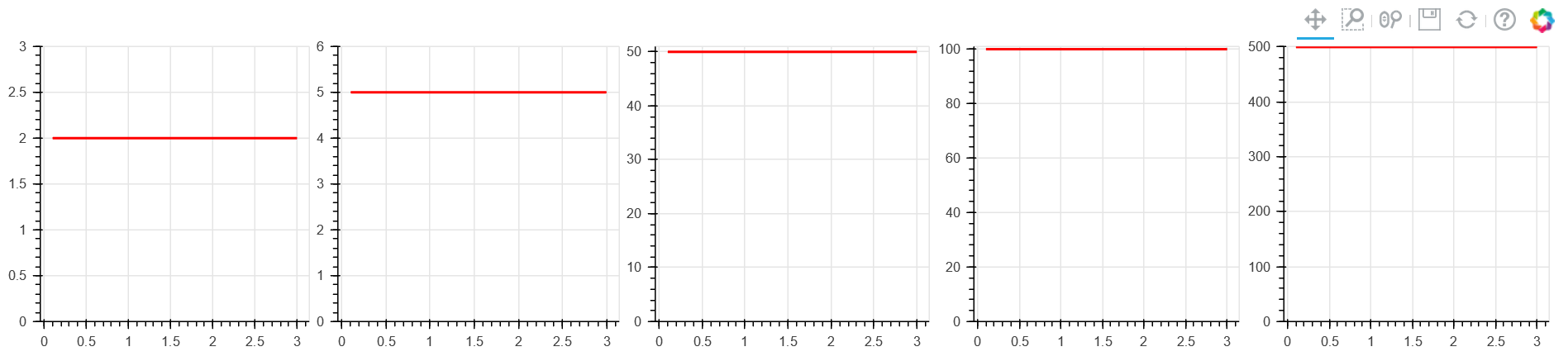What's wrong? Computed range for all lines is 0, yet for first few lines, where Y is relatively small, automated padding is added, but for line with Y=500 its too small.

###bryevdv commented Feb 26, 2020

 @Tikonderoga That's perfect I can run that and actually probe what the JS is doing. FYI I think you could just pass e.g. `y1=2` to the line call and not generate those lists.

###bryevdv commented Feb 26, 2020

 Looking at those now it looks possibly like the padding is always 1, which simple shows up very tiny on the scale of the last plot.

###Tikonderoga commented Feb 26, 2020

 Case 2: Auto-padding works correctly! ``````from bokeh.plotting import figure, output_file, show from bokeh.layouts import gridplot from bokeh.models import ColumnDataSource, Range1d, LabelSet, Label x = [0.1, 0.5, 1.0, 1.5, 2.0, 2.5, 3.0] y1 = [2 for xx in x] y1 = 3 y2 = [5 for xx in x] y2 = 7.5 y3 = [50 for xx in x] y3 = 55 y4 = [100 for xx in x] y4 = 150 y5 = [500 for xx in x] y5 = 750 p1 = figure(plot_width=300, plot_height=300) l1 = p1.line(x, y1, line_width=2, color='red') p2 = figure(plot_width=300, plot_height=300) l2 = p2.line(x, y2, line_width=2, color='red') p3 = figure(plot_width=300, plot_height=300) l3 = p3.line(x, y3, line_width=2, color='red') p4 = figure(plot_width=300, plot_height=300) l4 = p4.line(x, y4, line_width=2, color='red') p5 = figure(plot_width=300, plot_height=300) l5 = p5.line(x, y5, line_width=2, color='red') grid = gridplot([[p1, p2, p3, p4, p5], [None, None]], plot_width=250, plot_height=250) show(grid) ``````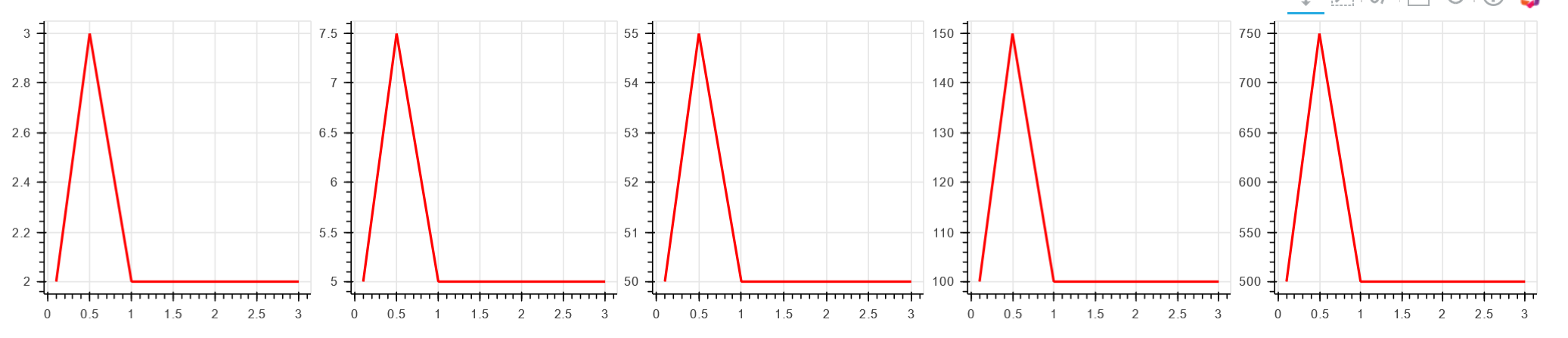Case 3 : Auto-padding broken with y_range.start = 0 ``````from bokeh.plotting import figure, output_file, show from bokeh.layouts import gridplot from bokeh.models import ColumnDataSource, Range1d, LabelSet, Label x = [0.1, 0.5, 1.0, 1.5, 2.0, 2.5, 3.0] y1 = [2 for xx in x] y1 = 3 y2 = [5 for xx in x] y2 = 7.5 y3 = [50 for xx in x] y3 = 55 y4 = [100 for xx in x] y4 = 150 y5 = [500 for xx in x] y5 = 750 p1 = figure(plot_width=300, plot_height=300) l1 = p1.line(x, y1, line_width=2, color='red') p1.y_range.start = 0 p2 = figure(plot_width=300, plot_height=300) l2 = p2.line(x, y2, line_width=2, color='red') p2.y_range.start = 0 p3 = figure(plot_width=300, plot_height=300) l3 = p3.line(x, y3, line_width=2, color='red') p3.y_range.start = 0 p4 = figure(plot_width=300, plot_height=300) l4 = p4.line(x, y4, line_width=2, color='red') p4.y_range.start = 0 p5 = figure(plot_width=300, plot_height=300) l5 = p5.line(x, y5, line_width=2, color='red') p5.y_range.start = 0 grid = gridplot([[p1, p2, p3, p4, p5], [None, None]], plot_width=250, plot_height=250) show(grid) ``````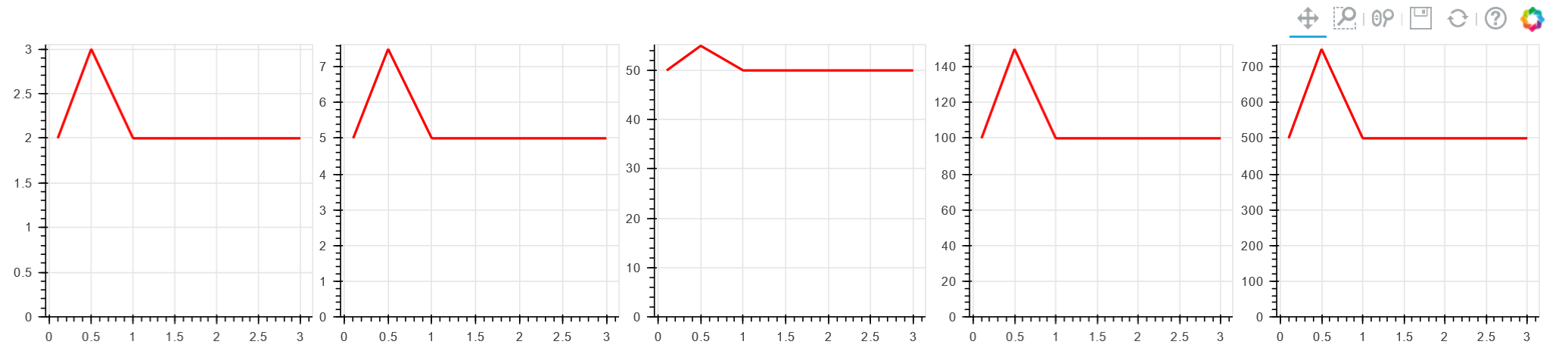That's an interesting one: all charts appears to be okay, even the last one, but one in the middle is not.

###Tikonderoga commented Feb 26, 2020

 Case 4: Playing with range_padding. Not working for straight lines! ``````from bokeh.plotting import figure, output_file, show from bokeh.layouts import gridplot from bokeh.models import ColumnDataSource, Range1d, LabelSet, Label x = [0.1, 0.5, 1.0, 1.5, 2.0, 2.5, 3.0] y1 = [500 for xx in x] p1 = figure(plot_width=300, plot_height=300) l1 = p1.line(x, y1, line_width=2, color='red') p1.y_range.start = 0 t1 = Label(x=20, y=100, x_units='screen', y_units='screen', text='No parameters set', render_mode='css', border_line_color='black', border_line_alpha=0, background_fill_color='white', background_fill_alpha=1.0, text_font_size = '8pt') p1.add_layout(t1) p2 = figure(plot_width=300, plot_height=300) l2 = p2.line(x, y1, line_width=2, color='red') p2.y_range.start = 0 p2.y_range.range_padding_units = 'percent' p2.y_range.range_padding = 0.1 t2 = Label(x=20, y=100, x_units='screen', y_units='screen', text="range_padding_units = percent\n range_padding = 0.1", render_mode='css', border_line_color='black', border_line_alpha=0, background_fill_color='white', background_fill_alpha=1.0, text_font_size = '8pt') p2.add_layout(t2) p3 = figure(plot_width=300, plot_height=300) l3 = p3.line(x, y1, line_width=2, color='red') p3.y_range.start = 0 p3.y_range.range_padding_units = 'percent' p3.y_range.range_padding = 10 t3 = Label(x=20, y=100, x_units='screen', y_units='screen', text="range_padding_units = percent\n range_padding = 10", render_mode='css', border_line_color='black', border_line_alpha=0, background_fill_color='white', background_fill_alpha=1.0, text_font_size = '8pt') p3.add_layout(t3) p4 = figure(plot_width=300, plot_height=300) l4 = p4.line(x, y1, line_width=2, color='red') p4.y_range.start = 0 p4.y_range.range_padding_units = 'absolute' p4.y_range.range_padding = 0 t4 = Label(x=20, y=100, x_units='screen', y_units='screen', text="range_padding_units = absolute\n range_padding = 0", render_mode='css', border_line_color='black', border_line_alpha=0, background_fill_color='white', background_fill_alpha=1.0, text_font_size = '8pt') p4.add_layout(t4) p5 = figure(plot_width=300, plot_height=300) l5 = p5.line(x, y1, line_width=2, color='red') p5.y_range.start = 0 p5.y_range.range_padding_units = 'absolute' p5.y_range.range_padding = 100 t5 = Label(x=20, y=100, x_units='screen', y_units='screen', text="range_padding_units = absolute\n range_padding = 100", render_mode='css', border_line_color='black', border_line_alpha=0, background_fill_color='white', background_fill_alpha=1.0, text_font_size = '8pt') p5.add_layout(t5) grid = gridplot([[p1, p2, p3, p4, p5], [None, None]], plot_width=250, plot_height=250) show(grid) ``````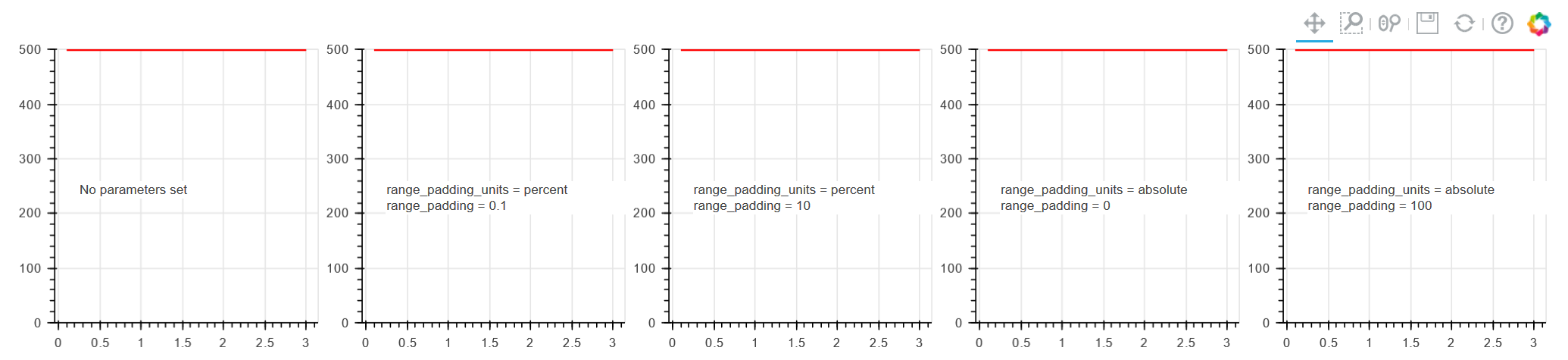If computed range for line is 0, manual padding not working at all.

###Tikonderoga commented Feb 26, 2020

 Case 5: manual padding with non-straight lines: ``````from bokeh.plotting import figure, output_file, show from bokeh.layouts import gridplot from bokeh.models import ColumnDataSource, Range1d, LabelSet, Label x = [0.1, 0.5, 1.0, 1.5, 2.0, 2.5, 3.0] y1 = [500 for xx in x] y1 = 550 p1 = figure(plot_width=300, plot_height=300) l1 = p1.line(x, y1, line_width=2, color='red') p1.y_range.start = 0 t1 = Label(x=20, y=100, x_units='screen', y_units='screen', text='No parameters set', render_mode='css', border_line_color='black', border_line_alpha=0, background_fill_color='white', background_fill_alpha=1.0, text_font_size = '8pt') p1.add_layout(t1) p2 = figure(plot_width=300, plot_height=300) l2 = p2.line(x, y1, line_width=2, color='red') p2.y_range.start = 0 p2.y_range.range_padding_units = 'percent' p2.y_range.range_padding = 0.1 t2 = Label(x=20, y=100, x_units='screen', y_units='screen', text="range_padding_units = percent\n range_padding = 0.1", render_mode='css', border_line_color='black', border_line_alpha=0, background_fill_color='white', background_fill_alpha=1.0, text_font_size = '8pt') p2.add_layout(t2) p3 = figure(plot_width=300, plot_height=300) l3 = p3.line(x, y1, line_width=2, color='red') p3.y_range.start = 0 p3.y_range.range_padding_units = 'percent' p3.y_range.range_padding = 10 t3 = Label(x=20, y=100, x_units='screen', y_units='screen', text="range_padding_units = percent\n range_padding = 10", render_mode='css', border_line_color='black', border_line_alpha=0, background_fill_color='white', background_fill_alpha=1.0, text_font_size = '8pt') p3.add_layout(t3) p4 = figure(plot_width=300, plot_height=300) l4 = p4.line(x, y1, line_width=2, color='red') p4.y_range.start = 0 p4.y_range.range_padding_units = 'absolute' p4.y_range.range_padding = 0 t4 = Label(x=20, y=100, x_units='screen', y_units='screen', text="range_padding_units = absolute\n range_padding = 0", render_mode='css', border_line_color='black', border_line_alpha=0, background_fill_color='white', background_fill_alpha=1.0, text_font_size = '8pt') p4.add_layout(t4) p5 = figure(plot_width=300, plot_height=300) l5 = p5.line(x, y1, line_width=2, color='red') p5.y_range.start = 0 p5.y_range.range_padding_units = 'absolute' p5.y_range.range_padding = 100 t5 = Label(x=20, y=100, x_units='screen', y_units='screen', text="range_padding_units = absolute\n range_padding = 100", render_mode='css', border_line_color='black', border_line_alpha=0, background_fill_color='white', background_fill_alpha=1.0, text_font_size = '8pt') p5.add_layout(t5) grid = gridplot([[p1, p2, p3, p4, p5], [None, None]], plot_width=250, plot_height=250) show(grid) ``````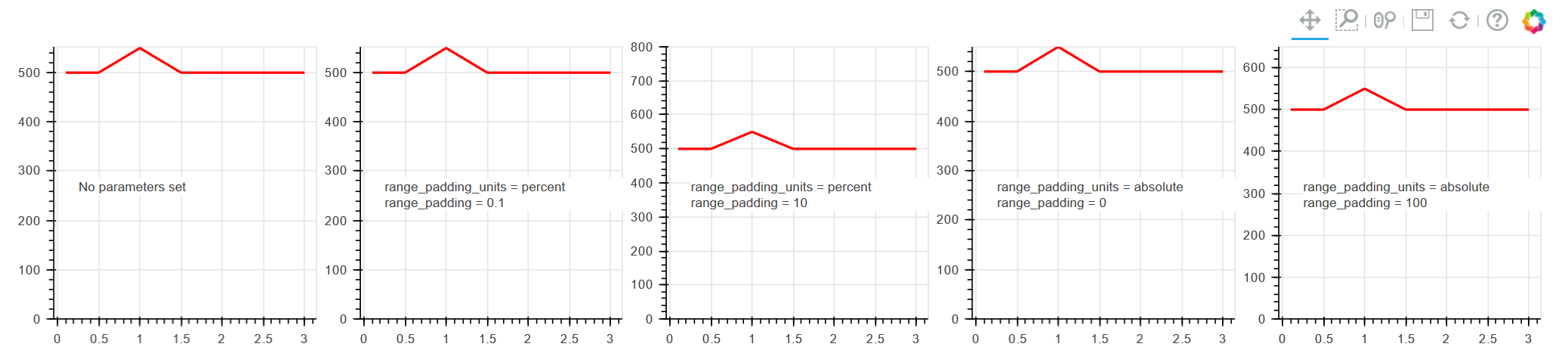Percentages are not really percentages? Setting padding to 10% increases Y range by 250 (middle chart) Padding in absolute units working!

###Tikonderoga commented Feb 26, 2020

 Case 6: range.only_visible = True, auto-padding not working when only 1 line is visible ``````from bokeh.plotting import figure, output_file, show from bokeh.layouts import gridplot from bokeh.models import ColumnDataSource, Range1d, LabelSet, Label x = [0.1, 0.5, 1.0, 1.5, 2.0, 2.5, 3.0] y1 = [500 for xx in x] y2 = [1000 for xx in x] y3 = [1500 for xx in x] legend_items = [] p1 = figure(plot_width=400, plot_height=400) l1 = p1.line(x, y1, line_width=2, color='red') l2 = p1.line(x, y2, line_width=2, color='blue') l3 = p1.line(x, y3, line_width=2, color='green') li1 = LegendItem(label='Line 1', renderers=[l1]) li2 = LegendItem(label='Line 2', renderers=[l2]) li3 = LegendItem(label='Line 3', renderers=[l3]) legend_items.append(li1) legend_items.append(li2) legend_items.append(li3) p1.y_range.only_visible = True legend = Legend(items=legend_items, location=(0, -30)) legend.click_policy = "hide" p1.add_layout(legend, 'left') p1.y_range.start = 0 show(p1) ``````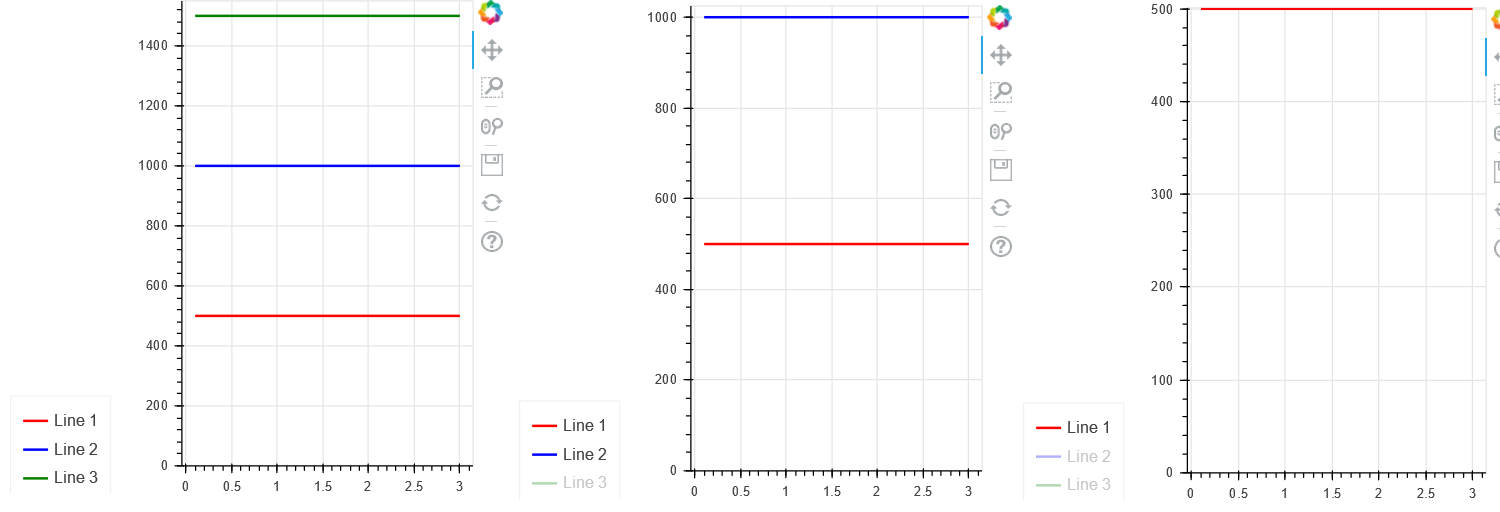For straight lines auto-padding stops working when only 1 line is visible.

###Tikonderoga commented Feb 26, 2020

 Case 7: same as case 6, but lines are not straight ``````from bokeh.plotting import figure, output_file, show from bokeh.layouts import gridplot from bokeh.models import ColumnDataSource, Range1d, LabelSet, Label x = [0.1, 0.5, 1.0, 1.5, 2.0, 2.5, 3.0] y1 = [500 for xx in x] y1 = 550 y2 = [1000 for xx in x] y2 = 1200 y3 = [1500 for xx in x] y3 = 1800 legend_items = [] p1 = figure(plot_width=400, plot_height=400) l1 = p1.line(x, y1, line_width=2, color='red') l2 = p1.line(x, y2, line_width=2, color='blue') l3 = p1.line(x, y3, line_width=2, color='green') li1 = LegendItem(label='Line 1', renderers=[l1]) li2 = LegendItem(label='Line 2', renderers=[l2]) li3 = LegendItem(label='Line 3', renderers=[l3]) legend_items.append(li1) legend_items.append(li2) legend_items.append(li3) p1.y_range.only_visible = True legend = Legend(items=legend_items, location=(0, -30)) legend.click_policy = "hide" p1.add_layout(legend, 'left') p1.y_range.start = 0 show(p1) ``````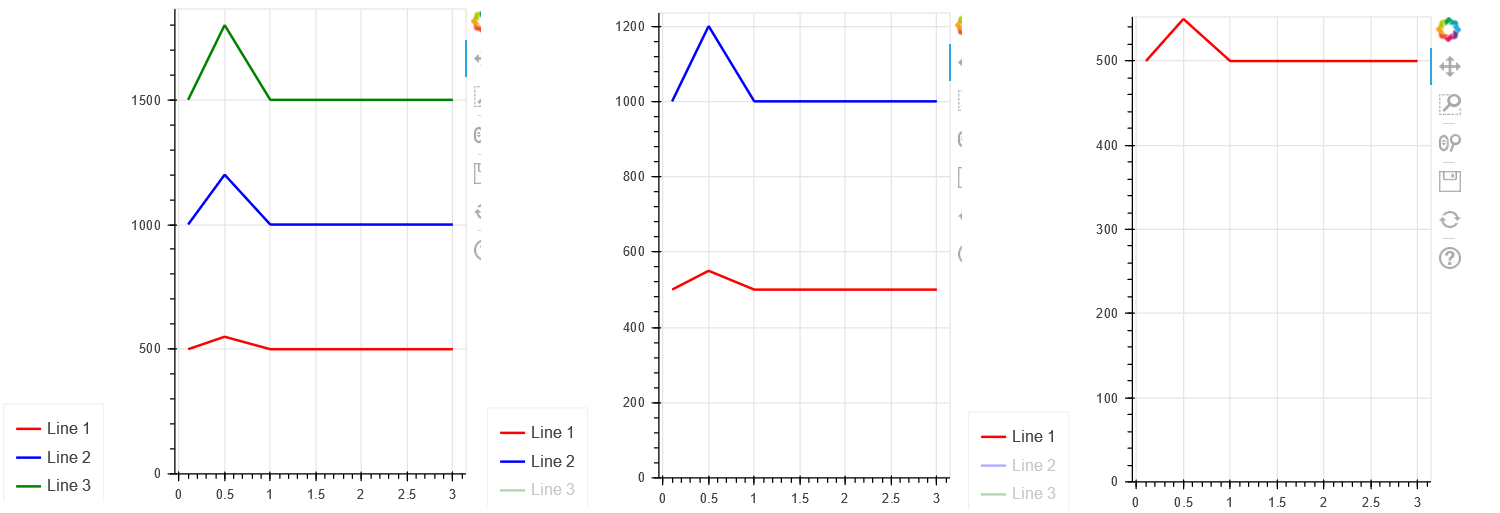Auto-padding not working for cases where only 1 line is visible even if line is not straight.

###Tikonderoga commented Feb 26, 2020

 Case 8: Manual padding, percentages ``````from bokeh.plotting import figure, output_file, show from bokeh.layouts import gridplot from bokeh.models import ColumnDataSource, Range1d, LabelSet, Label x = [0.1, 0.5, 1.0, 1.5, 2.0, 2.5, 3.0] y1 = [500 for xx in x] #y1 = 550 y2 = [1000 for xx in x] #y2 = 1200 y3 = [1500 for xx in x] #y3 = 1800 legend_items = [] p1 = figure(plot_width=400, plot_height=400) l1 = p1.line(x, y1, line_width=2, color='red') l2 = p1.line(x, y2, line_width=2, color='blue') l3 = p1.line(x, y3, line_width=2, color='green') li1 = LegendItem(label='Line 1', renderers=[l1]) li2 = LegendItem(label='Line 2', renderers=[l2]) li3 = LegendItem(label='Line 3', renderers=[l3]) legend_items.append(li1) legend_items.append(li2) legend_items.append(li3) p1.y_range.only_visible = True p1.y_range.range_padding_units = 'percent' p1.y_range.range_padding = 1 legend = Legend(items=legend_items, location=(0, -30)) legend.click_policy = "hide" p1.add_layout(legend, 'left') p1.y_range.start = 0 show(p1) ``````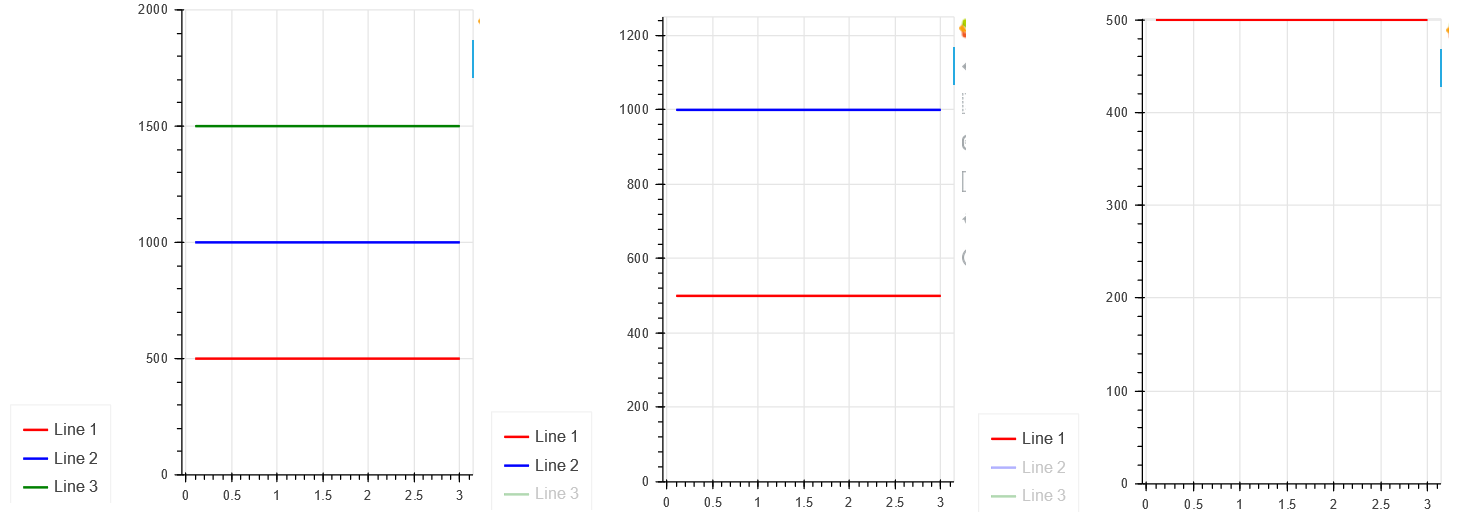This one also broken for case where 1 line is visible (probably because computed range for that case is 0).

###Tikonderoga commented Feb 26, 2020

 Case 9: Manul padding, percentages, not straight lines. ``````from bokeh.plotting import figure, output_file, show from bokeh.layouts import gridplot from bokeh.models import ColumnDataSource, Range1d, LabelSet, Label x = [0.1, 0.5, 1.0, 1.5, 2.0, 2.5, 3.0] y1 = [500 for xx in x] y1 = 550 y2 = [1000 for xx in x] y2 = 1200 y3 = [1500 for xx in x] y3 = 1800 legend_items = [] p1 = figure(plot_width=400, plot_height=400) l1 = p1.line(x, y1, line_width=2, color='red') l2 = p1.line(x, y2, line_width=2, color='blue') l3 = p1.line(x, y3, line_width=2, color='green') li1 = LegendItem(label='Line 1', renderers=[l1]) li2 = LegendItem(label='Line 2', renderers=[l2]) li3 = LegendItem(label='Line 3', renderers=[l3]) legend_items.append(li1) legend_items.append(li2) legend_items.append(li3) p1.y_range.only_visible = True p1.y_range.range_padding_units = 'percent' p1.y_range.range_padding = 1 legend = Legend(items=legend_items, location=(0, -30)) legend.click_policy = "hide" p1.add_layout(legend, 'left') p1.y_range.start = 0 show(p1) ``````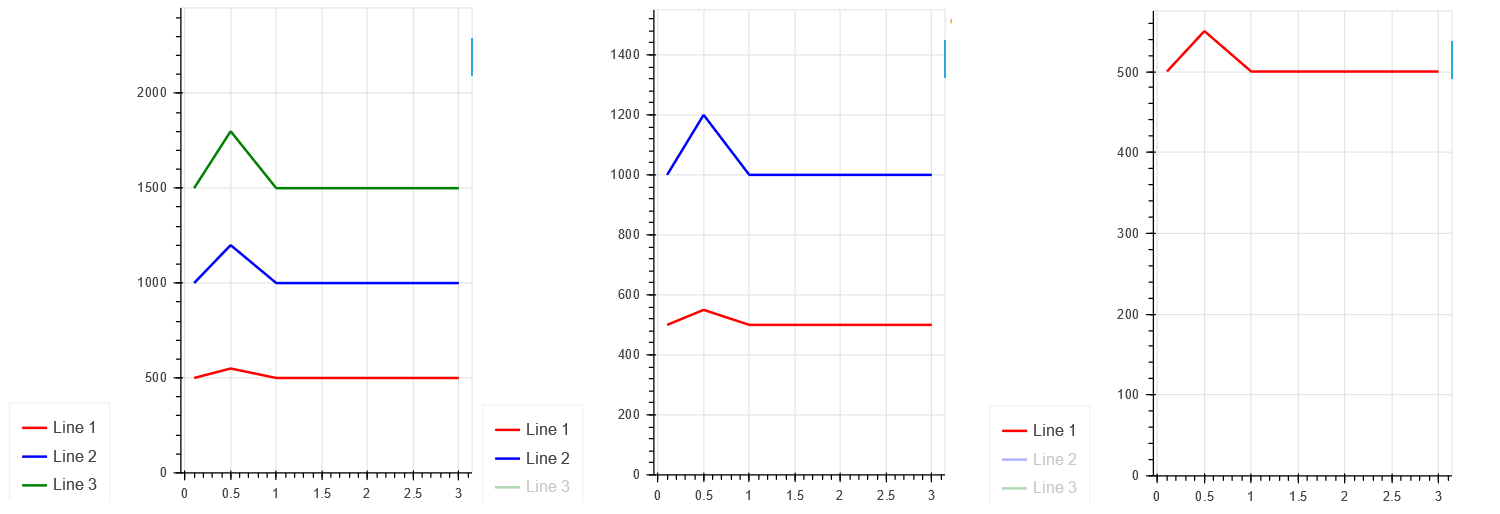Finally, we have padding in all cases. But it's not perfect (it's too large for a case when all 3 lines are visible).

###Tikonderoga commented Feb 26, 2020 • edited

 Overall, I think computed min/max range should acknowledge the fact what start or end of the range is pinned. For example, is computed range for line is (Ymix:Ymax), but y_range.start = Ys, computed range should be (Ys:Ymax) or even (min(Ymin, Ys): Y:max). I think it would solve all the issues above.

###mattpap commented Feb 26, 2020 • edited

 Overall, I think computed min/max range should acknowledge the fact what start or end of the range is pinned. Currently the implementation completely ignores explicitly set `start` and `end` values when computing range padding. This should be a fairly easy fix. If you would like to take a stab at it, then you will have to modify this method to respect `_initial_start` and `_initial_end` values.

###Tikonderoga commented Feb 28, 2020

 @mattpap @bryevdv I'm trying to build a fix and verify it before creating pull request. I've managed to build Bokeh locally, but when I'm trying to run tests in notebook, I'm seeing following error: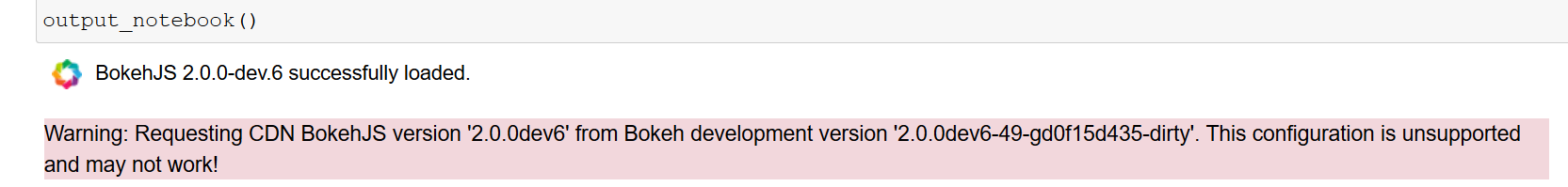How would I fix that? Should I go to Discourse with such questions?

###mattpap commented Feb 28, 2020

 My personal approach to this is as follows: terminal 1: `BOKEH_DEV=true bokeh static` terminal 2: `BOKEH_RESOURCES=server-dev jupyter-lab` This will make sure that you always have the most recently built bundles and debugging experience in jlab is the most efficient. Another personal preference is not to use jlab for bokeh development, as it's usually more trouble than it's worth.

###bryevdv commented Feb 28, 2020

 `BOKEH_RESOURCES=inline` is another option, but also agree: if you are not working on something that is specifically jupyter-related, then working there adds quite a lot of (IMO) otherwise unnecessary complication.

###Tikonderoga commented Feb 28, 2020

 Thanks, ``````output_notebook(resources=INLINE) `````` does the trick.

###Tikonderoga commented Feb 28, 2020

 And follow-up question: is there any instructions how to contribute to bokeh source code? Or is it just "create branch -> test -> create pull request" routine?

###bryevdv commented Feb 28, 2020

 @Tikonderoga yes exactly PRs from forks are the standard way to proceed

added a commit that referenced this issue Apr 7, 2020
``` Add tests for issues #9522, #9703 and #9724 ```
``` 28506c9 ```
added a commit that referenced this issue Apr 7, 2020
``` Add tests for issues #9522, #9703 and #9724 (#9891) ```
``` f48bb93 ```
```* Add tests for issues #9522, #9703 and #9724

* Reorganize Figure.Attrs under a namespace

* Better filtering in devtools' test runner

Use e.g. http://localhost:5777/integration/run?k=Bug.